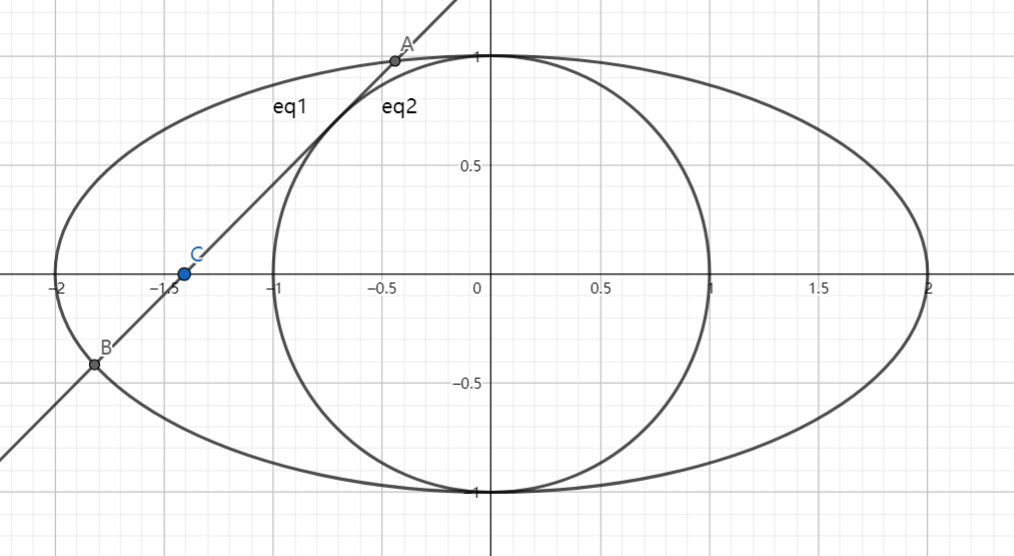# SAT1000 - P808

Geometry Level pendingAs shown above, the ellipse has equation: $\dfrac{x^2}{4}+y^2=1$.

If line $l$ passes through point $C(m,0)$ and is tangent to circle: $x^2+y^2=1$.

$l$ intersects with the ellipse at point $A,B$, then find the maximum value of $|AB|$.

Let $M$ be the maximum value. Submit $\lfloor 1000M \rfloor$.

Have a look at my problem set: SAT 1000 problems

×